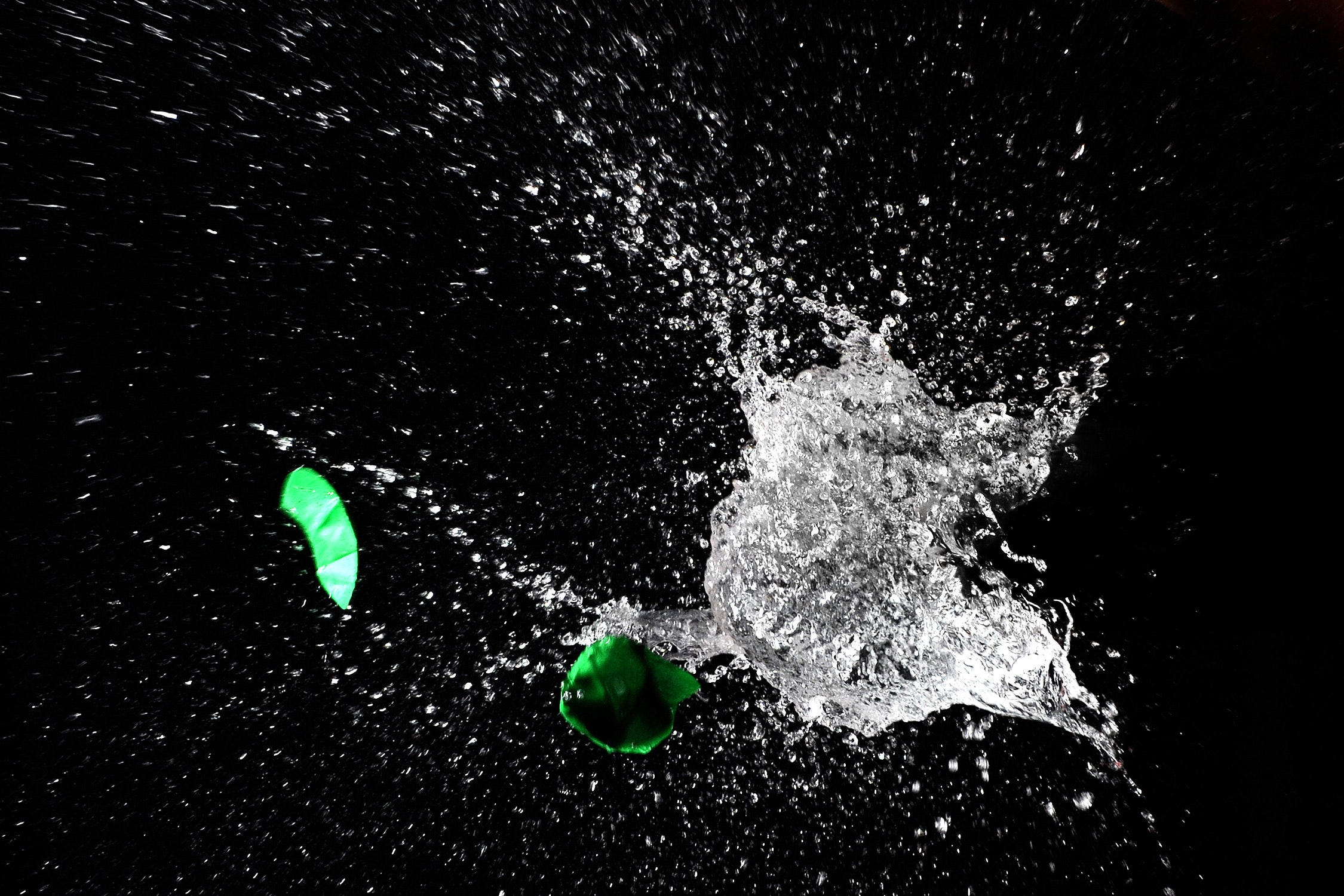# Solution of Physics Numericals for class 9 (set 1 Q15)

Last updated on November 6th, 2020 at 09:23 am

## Problem statement

A force causes an acceleration of 10 m/s^2 in a body of mass 500 g. What acceleration would be caused by the same force in a body of mass 5 kg.

Solution

Case 1
mass = m=500 g = 0.5 kg

Acceleration = a =10 m/s^2

Force = ma = 0.5 . 10 = 5 N

This force value will be used as input parameter in the next case.

Case 2

Mass = m=5 kg

Force = F=5 N

And the acceleration = a (to be found out)
F = ma
=> a = F /m = 5/5 m/s^2 = 1 m/s^2

See also  Solution to problems - class 9 - Set 1 Q34
Scroll to top
error: physicsTeacher.in